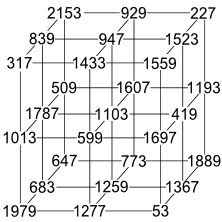# 2153

This number is a prime.The largest prime number for which R(n)+6 is a probable prime - unfortunately R2153 itself has the factor 12919.Suzuki magic cube
In 1977, Akio Suzuki used primes ranging from 53 to 2153 to construct the magic cube with smallest possible magic sum (for cubes with side three and distinct prime entries). [Heinz]2153 and the next prime are the smallest consecutive primes that have the same sum of digits as each other's prime indices. [Gaydos]